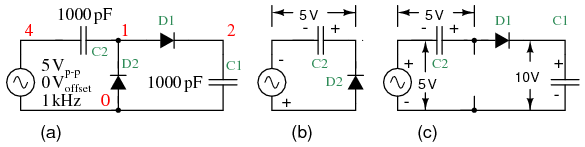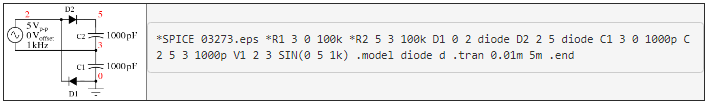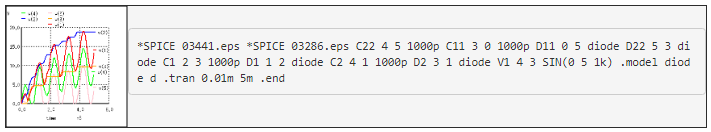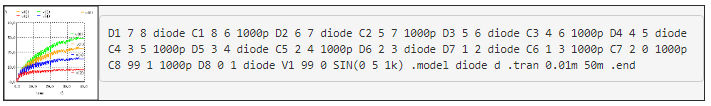# 3.08: Voltage Multipliers (Doublers, Triplers, Quadruplers, and More)

We’ll first go over several types of voltage multipliers—voltage doubler (half- and full-wave), voltage tripler, and voltage quadrupler—then make some general notes about voltage multiplier safety and finish up with the Cockcroft-Walton multiplier.

## Voltage Doubler

A voltage doubler application is a DC power supply capable of using either a 240 VAC or 120 VAC source. The supply uses a switch selected full-wave bridge to produce about 300 VDC from a 240 VAC source. The 120 V position of the switch rewires the bridge as a doubler producing about 300 VDC from the 120 VAC. In both cases, 300 VDC is produced. This is the input to a switching regulator producing lower voltages for powering, say, a personal computer.

## Half-Wave Voltage Doubler

The half-wave voltage doubler in Figure below (a) is composed of two circuits: a clamper at (b) and peak detector (half-wave rectifier) in Figure prior, which is shown in modified form in Figure below (c). C2 has been added to a peak detector (half-wave rectifier).Half-wave voltage doubler (a) is composed of (b) a clamper and (c) a half-wave rectifier.

Referring to Figure above (b), C2 charges to 5 V (4.3 V considering the diode drop) on the negative half cycle of AC input. The right end is grounded by the conducting D2. The left end is charged at the negative peak of the AC input. This is the operation of the clamper.

During the positive half cycle, the half-wave rectifier comes into play at Figure above (c). Diode D2 is out of the circuit since it is reverse biased. C2 is now in series with the voltage source. Note the polarities of the generator and C2, series aiding. Thus, rectifier D1 sees a total of 10 V at the peak of the sinewave, 5 V from generator and 5 V from C2. D1 conducts waveform v(1) (Figure below), charging C1 to the peak of the sine wave riding on 5 V DC (Figure below v(2)). Waveform v(2) is the output of the doubler, which stabilizes at 10 V (8.6 V with diode drops) after a few cycles of sinewave input.### Full-Wave Voltage Doubler

The full-wave voltage doubler is composed of a pair of series stacked half-wave rectifiers. (Figure below) The corresponding netlist is in Figure below. The bottom rectifier charges C1 on the negative half cycle of input. The top rectifier charges C2 on the positive halfcycle. Each capacitor takes on a charge of 5 V (4.3 V considering diode drop). The output at node 5 is the series total of C1 + C2 or 10 V (8.6 V with diode drops).Full-wave voltage doubler consists of two half-wave rectifiers operating on alternating polarities.

Note that the output v(5) Figure below reaches full value within one cycle of the input v(2) excursion.Full-wave voltage doubler: v(2) input, v(3)voltage at mid point, v(5) voltage at output

Figure below illustrates the derivation of the full-wave doubler from a pair of opposite polarity half-wave rectifiers (a). The negative rectifier of the pair is redrawn for clarity (b). Both are combined at (c) sharing the same ground. At (d) the negative rectifier is re-wired to share one voltage source with the positive rectifier. This yields a ±5 V (4.3 V with diode drop) power supply; though, 10 V is measurable between the two outputs. The ground reference point is moved so that +10 V is available with respect to ground.Full-wave doubler: (a) Pair of doublers, (b) redrawn, (c) sharing the ground, (d) share the same voltage source. (e) move the ground point.

## Voltage Tripler

A voltage tripler (Figure below) is built from a combination of a doubler and a half wave rectifier (C3, D3). The half-wave rectifier produces 5 V (4.3 V) at node 3. The doubler provides another 10 V (8.4 V) between nodes 2 and 3. for a total of 15 V (12.9 V) at the output node 2 with respect to ground. The netlist is in Figure below.Voltage tripler composed of doubler stacked atop a single stage rectifier.

Note that V(3) in Figure below rises to 5 V (4.3 V) on the first negative half cycle. Input v(4) is shifted upward by 5 V (4.3 V) due to 5 V from the half-wave rectifier. And 5 V more at v(1) due to the clamper (C2, D2). D1 charges C1 (waveform v(2)) to the peak value of v(1).Voltage tripler: v(3) half-wave rectifier, v(4) input+ 5 V, v(1) clamper, v(2) final output.

A voltage quadrupler is a stacked combination of two doublers shown in Figure below. Each doubler provides 10 V (8.6 V) for a series total at node 2 with respect to ground of 20 V (17.2 V).

The netlist is in Figure below.Voltage quadrupler, composed of two doublers stacked in series, with output at node 2.

The waveforms of the quadrupler are shown in Figure below. Two DC outputs are available: v(3), the doubler output, and v(2) the quadrupler output. Some of the intermediate voltages at clampers illustrate that the input sinewave (not shown), which swings by 5 V, is successively clamped at higher levels: at v(5), v(4) and v(1). Strictly v(4) is not a clamper output. It is simply the AC voltage source in series with the v(3) the doubler output. None the less, v(1) is a clamped version of v(4)Voltage quadrupler: DC voltage available at v(3) and v(2). Intermediate waveforms: Clampers: v(5), v(4), v(1).

## Notes on Voltage Multipliers and Line Driven Power Supplies

Some notes on voltage multipliers are in order at this point. The circuit parameters used in the examples (V= 5 V 1 kHz, C=1000 pf) do not provide much current, microamps. Furthermore, load resistors have been omitted. Loading reduces the voltages from those shown. If the circuits are to be driven by a kHz source at low voltage, as in the examples, the capacitors are usually 0.1 to 1.0 µF so that milliamps of current are available at the output. If the multipliers are driven from 50/60 Hz, the capacitor are a few hundred to a few thousand microfarads to provide hundreds of milliamps of output current. If driven from line voltage, pay attention to the polarity and voltage ratings of the capacitors.

Finally, any direct line driven power supply (no transformer) is dangerous to the experimenter and line operated test equipment. Commercial direct driven supplies are safe because the hazardous circuitry is in an enclosure to protect the user. When breadboarding these circuits with electrolytic capacitors of any voltage, the capacitors will explode if the polarity is reversed. Such circuits should be powered up behind a safety shield.

## Cockcroft-Walton Multiplier

A voltage multiplier of cascaded half-wave doublers of arbitrary length is known as a Cockcroft-Walton multiplier as shown in Figure below. This multiplier is used when a high voltage at low current is required. The advantage over a conventional supply is that an expensive high voltage transformer is not required– at least not as high as the output.Cockcroft-Walton x8 voltage multiplier; output at v(8).

The pair of diodes and capacitors to the left of nodes 1 and 2 in Figure above constitute a half-wave doubler. Rotating the diodes by 45o counterclockwise, and the bottom capacitor by 90o makes it look like Figure prior (a). Four of the doubler sections are cascaded to the right for a theoretical x8 multiplication factor. Node 1 has a clamper waveform (not shown), a sinewave shifted up by 1x (5 V). The other odd numbered nodes are sinewaves clamped to successively higher voltages. Node 2, the output of the first doubler, is a 2x DC voltage v(2) in Figure below. Successive even numbered nodes charge to successively higher voltages: v(4), v(6), v(8)Cockcroft-Walton (x8) waveforms. Output is v(8).

Without diode drops, each doubler yields 2Vin or 10 V, considering two diode drops (10-1.4)=8.6 V is realistic. For a total of 4 doublers one expects 4·8.6=34.4 V out of 40 V.

Consulting Figure above, v(2) is about right; however, v(8) is <30 V instead of the anticipated 34.4 V. The bane of the Cockcroft-Walton multiplier is that each additional stage adds less than the previous stage. Thus, a practical limit to the number of stages exist. It is possible to overcome this limitation with a modification to the basic circuit. [ABR] Also note the time scale of 40 msec compared with 5 ms for previous circuits. It required 40 msec for the voltages to rise to a terminal value for this circuit. The netlist in Figure above has a “.tran 0.010m 50m” command to extend the simulation time to 50 msec; though, only 40 msec is plotted.

The Cockcroft-Walton multiplier serves as a more efficient high voltage source for photomultiplier tubes requiring up to 2000 V. [ABR] Moreover, the tube has numerous dynodes, terminals requiring connection to the lower voltage “even numbered” nodes. The series string of multiplier taps replaces a heat generating resistive voltage divider of previous designs.

An AC line operated Cockcroft-Walton multiplier provides high voltage to “ion generators” for neutralizing electrostatic charge and for air purifiers.

## Voltage Multiplier Review:

• A voltage multiplier produces a DC multiple (2,3,4, etc) of the AC peak input voltage.
• The most basic multiplier is a half-wave doubler.
• The full-wave double is a superior circuit as a doubler.
• A tripler is a half-wave doubler and a conventional rectifier stage (peak detector).
• A quadrupler is a pair of half-wave doublers
• A long string of half-wave doublers is known as a Cockcroft-Walton multiplier.